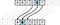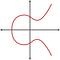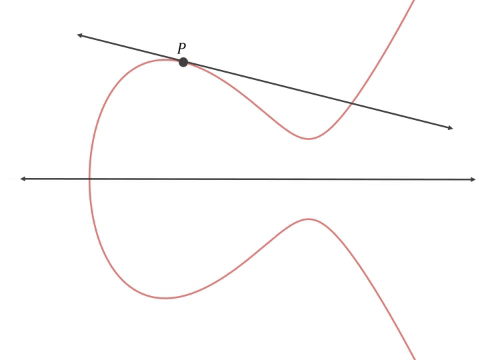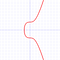# Elliptic-Curve Cryptography

## The Curves That Keep The Bitcoin Secure

This isn’t an easy subject but it is one that has wide ranging applications and is therefore worth putting in the effort to understand. It has enabled a reduction in key generation time and key weight over first generation public key systems like prime factorization cryptography, as well as a 10-fold increase in security and it serves as the basis of much of the public key cryptography in today’s cryptocurrency ecosystem. So let’s break it down so we can trust it.

Discover and review best Crypto softwares

## Hey Alice, pass the key.

First, let’s rewind. Say you want to send a message to someone and you don’t want anyone to read it but that person. So you “encrypt” it in some way that someone cannot read it unless they know how to “decrypt” it. This can be done with a simple jumbling or shifting of the letters in a known way. One of the oldest encryptions known is the Cesar Cipher, does just that. By shifting the letters some number of places X, the words in your message become unreadable [Fig.1]. Once X is communicated, the jumble of letters can be unshifted to decrypt the message.Fig. 1 A Cesar Cipher where the shift is 3. “E” will then take the place of “B”, “F” for “C” and so on.

There are two significant issues with this method. First, you have to somehow tell the person that you wish to communicate with, the secret key to break the code and trust them to keep it safe. And second, in this age of computers, this is not very hard to break.

We could come up with another scheme of encrypting the data like using a specific page in a book as the cipher, but in every case I can think of, we need some safe way to communicate to another person the key. How would this be done if we were far away? Communicate through a digital format or trust a third party to share the secret? We have to find another way.

## Public-Key Cryptography

In comes public key (or asymmetric) cryptography. Pioneered by Whit Diffie and Martin Hellman in the late 1970s, public key cryptography uses pairs of keys; one public, which can be known to all, and one private, which is kept secret. This system enables anyone to encrypt a message using the receiver’s public key and that message can then only be decrypted with the receiver’s private key.

A public key is a key which can lock the door but cannot unlock it.

This revolutionary step solved the issue of having to share a secret in order to decrypt the message and also enabled the authentication of messages encrypted by the owner of a public key.

These key-pairs rely on “Trapdoor Functions” which are easy to compute in one direction but much harder to reverse. Based on mathematics, the strength of these key-pairs rely on the computational effort that would have to be expended in order to derive the private key from the public key.

## RSA

In one of the first widely used public-key crytposystems, RSA (Rivest–Shamir–Adleman), you rely on the fact that multiplying large numbers together is easy and factoring them is hard.

To create an RSA key-pair (without going into too much detail), you select two large prime numbers, multiply them together to get a maximum value (max or MOD, meaning the number where the clock resets to 0), and select one of the primes to be the public key, pub, and use the other prime number to derive your private key, priv. With these three numbers someone can multiply their message by pub, wrap around any numbers that go above max, and the corresponding jumbled mess of numbers can be multiplied by priv to yield the original data. I know it sounds hand-wavey but it’s true (and if you want to see it done simply, take a look at the links at the bottom of this article). It’s kind of like if I hid the hour hand on the clock and rotated the minute hand around it several times then showed it to you. It may say 45 minutes but you don’t know how many times it went around the clock before it landed there. Only I would know.

The take away is that using this mathematics, you can find 2 numbers such that when you multiply a number by itself a number of times and get a random-looking number, you can then multiply it by itself again a secret number of times to get the original number back.

The only problem with this is that factoring is a very popular problem and one that is not that very difficult to do on a bit by bit basis. With the advance of computational speed and increased efficiency in factoring algorithms, the prime numbers in the RSA key-pair generation have to continually grow in order to outpace technology and maintain security. This system has therefore become unsustainable and not applicable in mobile or low power situations. Another, more robust, Trapdoor Function had to be found.

## Elliptic Curves

This real world use case of mathematics invigorated the research into more fringe mathematics in the effort to find something that would further revolutionize cryptography.

In 1985, cryptography based on elliptic curves was proposed independently by Neal Koblitz and Victor Miller.

Elliptic curves have some curious characteristics that make them useful. They are defined as a curve that is completely smooth (non-singular) and a line between two points on this curve will always intersect a third point (projective) [Fig. 2]. This allows you to quickly hop around this curve easily (computationally) and procedurally come up with an endpoint that has seemingly no relation to the starting point and is very difficult to reverse the path that led you there.Fig. 2 Elliptical curve with points defined as y² = x³ + ax + b

In the cryptographic usage, the same ideas of finding two unique numbers (points in a two dimensional curve) which are related and a max ceiling to wrap around apply.

How do we go about getting two numbers that are related but in a way that no one can tell? Well we use the curve’s projective property and draw a line tangent to the starting point P, finding where it intersects the curve at a second point Pʹ. Then flip the axis and draw a line from that new point (2•P) through the starting point and find the new intersection point Pʹʹ. Then flip the axis and draw a line from that new point (3•P) through the starting point and find the new intersection point Pʹʹʹ etc. (this mathematical operation is called point multiplication) [Fig. 3].Fig. 3 Point multiplication starting with the tangent to point P and ending with point 3•P

We do this n times and end up with a point on the curve, Q, that has no obvious relationship to the starting point and can be defined as Q=n•P where n is the number of iterations of point multiplication done. Mathematically this works out to Q = P + P + P … n times.

So if you knew the curve we used, the starting point P and the ending point Q, could you determine n? It turns out there is no known algorithm to do this. No short cuts in determining how many times you “dotted” P in order to get to Q. You basically have to just keep adding P to itself and count how many times you have to do it in order to get to Q (or the other way around). This is fairly easy with small n, but what happens when n gets big? and I mean really big…

## Elliptic Curves in Application

The elliptic curve used by Bitcoin, Ethereum and many others is the secp256k1 curve, with a equation of y² = x³+7 and looks like this:Fig. 4 Elliptic curve secp256k1 over real numbers. Note that the real implementation of the curve is over a defined prime field of positive integers and therefore looks nothing like the above.

And has a defined starting point used by all key generation, P(x, y), with x and y coordinates:

x-coordinate: 55066263022277343669578718895168534326250603453777594175500187360389116729240

y-coordinate:
32670510020758816978083085130507043184471273380659243275938904335757337482424

As you can see with a starting point THAT big, having an ending point larger than the allowed 512-bit key size is very possible. We therefore have to set a maximum value that we wrap around to establish a field of allowed points that fit our key size.

For this specific curve the maximum (mod) value is defined by a prime number (to yield a prime field) of:

OR

115792089237316195423570985008687907853269984665640564039457584007908834671663

OR

115 quattuorvigintillion …

OR

.12% of all the atoms in the known universe.

# aka real big.

As negative or non-integer values are difficult to use in computation, we only want to use the integer points in the curve with negative values inversed and use the defined maximum value to wrap values back to 0 as to prevent getting values too large. We end up with something that looks less like a curve, but more like a random smattering of dots, although some symmetry can be seen [Fig. 5].

So now with this newly defined “curve”, we do the point multiplication from our new starting point, we wrap around our modulo much like the minute hand going around a clock, and after n times we end on point Q [or A•B = C as shown in Fig. 6].

Since the number of available points is linearly related to the field size (number of points = Fᵖ +1), and since there is no easy way to find n given P and Q, you just have to go through the whole list, trying 115792089237316195423570985008687907853269984665640564039457584007908834671663–1 possibilities to find it (with each one being just as possible as another). So it therefore makes sense to make Q the public key and n the private key with everyone using the same curve and starting point, P.

So in the end, your private key is just a large integer and your public key is a point on the curve corresponding to your private key point multiplied with the starting point.

## How “Secure” is Secure?

Let’s try to visualize just how secure this is. If the selection of n was truly random, how long would it take for you to find a specific n, or just in general, any “collision” with another, in-use, private key? Lets say you could try 250 billion billion possibilities a second, 5 times the current Bitcoin network hash rate, it would still take 1⁰¹⁰ times the age of the universe to find a match. Basically anything else happening is more probable than finding a match.

There has been some research done to establish ways of measuring the security of these encryption types. In these scales, ECC in the defined curve and field above is considered “Globally Secure”, meaning the energy a computer, or group of computers, would have to use in order to find a specific n would be enough energy to boil 1,400,000,000 km³ of water, which is just about all the water on the surface of the earth. That’s probably secure enough.

## How does this apply to the addresses we see today?

Obviously the ways we are used to seeing addresses, being large strings of both numbers and letters, are different than a number and points on a curve. That’s because they are hashed in such a way to reduce the size of the string for light weight ease of use. In Bitcoin, the coordinates of Q are combined and put through a cascade of hashing algorithms designed to compress the key and add a validity checksum for address checking [Fig. 7].

So hopefully after all that you now understand the ideas behind elliptic curve cryptography a bit better and can trust the security of your cryptocurrencies and private communications to the statistics of cosmically large numbers.

## Coinmonks

### By Coinmonks

A newsletter that brings you week's best crypto and blockchain stories and trending news directly in your inbox, by CoinCodeCap.com Take a look

Written by

## Grayblock

#### Blockchain and IoT Systems Integrators